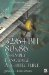Min -- MinimumMin Minimum

 MMX SSE SSE2 3D Now 3DMX+ 8—8-bit 4—16-bit 4—SPFP 1—SPFP 16—8-bit 8—16-bit 2—DPFP 1—DPFP 2—SPFP 8—8-bit 4—16-bit

The simplified form of this parallel instruction individually compares the integer or floating-point source arguments and returns the minimum value result in the destination.

vD[] = (vA[] < vB[])  ?  vA[] : vB[] // an element

Pseudo Vec

The previous C equation is a branching equation, which can cause a processor misprediction whether the branch is taken or not. A scalar operation could be done with branchless code such as follows :

// r=(p < q) ? p : q; __inline MIN(int p, int q) {    r = (pq) >> INT_MAX_BITS;         // ()=0xFFFFFFFF (+)=0x00000000    return (p & r)  (q & (r^1));     // keep lower of p or q }

The two values p and q are being compared so that the retained value is the smaller one. If p is less than q, subtraction (pq) generates a negative value. The sign bit is then arithmetically shifted to the right the size of the data word, which would be a 31-bit shift and thus latching the MSB of 1 into all the bits. If p = q, then pq is positive, the sign bit of zero would be latched into all the bits, thus generating a mask of all zeros. By bit blending with the mask and its inverse, the resulting value will be retained. For legacy processors that do not support this instruction it can be replicated in parallel using a packed arithmetic shift right or with a packed compare, if they are supported.

N 8-Bit Integer Minimum

Mnemonic

P

PII

K6

3D!

3Mx+

SSE

SSE2

A64

SSE3

E64T

PMINUB32/64-Bit 80x86 Assembly Language Architecture
ISBN: 1598220020
EAN: 2147483647
Year: 2003
Pages: 191

Similar book on Amazon